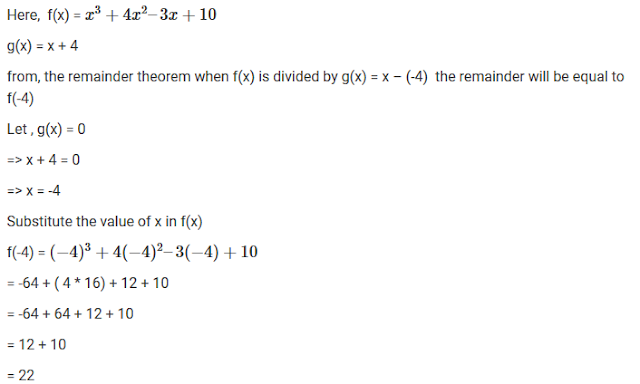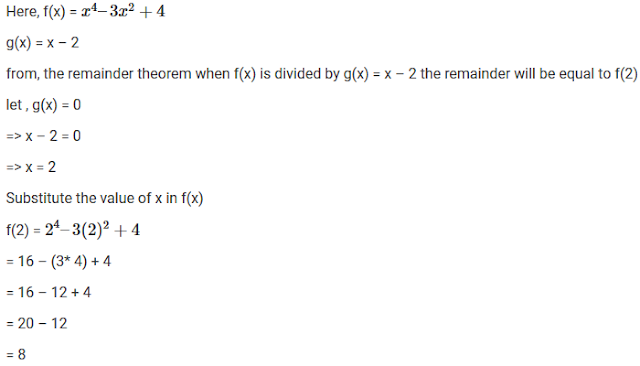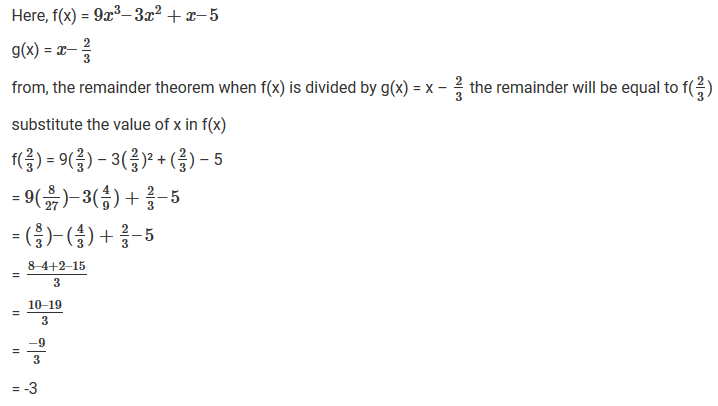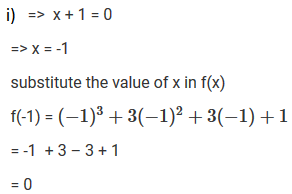#### Chapter 6 Factorization of Polynomials R.D. Sharma Solutions for Class 9th Math Exercise 6.3

Exercise 6.3

1. In each of the following, using the remainder theorem, find the remainder when f(x) is divided by g(x) and verify the result by actual division: (1−8).
(i) f(x) =x3+4x2-3x+10, g(x) = x+4

Solution2.  f(x) = 4x4-3x3-2x2+x-7, g(x) = x-1

Solution3.  f(x) = 2x4-6x3+2x2-x+2, g(x) = x+2

Solution4.  f(x) = 4x2-12x2+14x-3, g(x) = 2x-1

Solution5.  f(x) = x3-6x2+2x-4, g(x) = 1-2x

Solution6.  f(x) = x4-3x2+4, g(x) = x-2

Solution7.  f(x) = 9x3-3x2+x-5, g(x) = x - 2/3

Solution8. f(x) = 3x4+2x3x2/3 - x/9 + 2/27, g(x) = x + 2/3

Solution9. If the polynomials 2x3+ax2+3x-5 and x3+x2-4x+a leave the same remainder when divided by x-2, find the value of a.

Solution10.  The polynomials ax3+3x2-3 and 2x3+5x+a when divided by (x-4) leave the remainders R1 and R2 respectively. Find the value of a in each of the following cases, if
(a) R1 = R2
(b) R1+R2 =0
(c) 2R1 -R2 =0

Solution11.  If the polynomials ax3+3x2- 13 and 2x3-5x+a, when divided by (x-2) leave the same remainder, find the value of a.

SolutionSolution